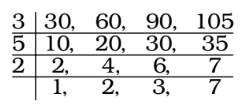## LCM and HCF

#### LCM and HCF

1. 4 bells ring at intervals of 30 minutes, 1 hour, 11/2 hour and 1 hour 45 minutes respectively. All the bells ring simultaneously at 12 noon. They will again ring simultaneously at :

1.  1 1 hours = 90 minutes 2

1 hour and 45 minutes = 105 minutes
1 hour = 60 minutes
∴ LCM of 30 minutes, 60 minutes, 90 minutes and 105 minutes∴ LCM = 3 × 5 × 2 × 2 × 3 × 7 = 1260 minutes
 1260 minutes = 1260 = 21 hours 60

##### Correct Option: D

 1 1 hours = 90 minutes 2

1 hour and 45 minutes = 105 minutes
1 hour = 60 minutes
∴ LCM of 30 minutes, 60 minutes, 90 minutes and 105 minutes∴ LCM = 3 × 5 × 2 × 2 × 3 × 7 = 1260 minutes
 1260 minutes = 1260 = 21 hours 60

∴ The bell will again ring simultaneously after 21 hours.
∴ Time will be = 12 noon + 21 hours = 9 a.m.
Required Time will be 9 a.m.

1. Four bells ring at intervals of 4, 6, 8 and 14 seconds. They start ringing simultaneously at 12.00 O’clock. At what time will they again ring simultaneously ?

1. LCM of 4, 6, 8, 14 = 168 seconds = 2 minutes 48 seconds
They ring again at 12 + 2 min. 48 sec.

##### Correct Option: A

LCM of 4, 6, 8, 14 = 168 seconds = 2 minutes 48 seconds
They ring again at 12 + 2 min. 48 sec.
Required time = 12 hrs. 2 min. 48 sec.

1. Four runners started running simultaneously from a point on a circular track. They took 200 seconds, 300 seconds, 360 seconds and 450 seconds to complete one round. After how much time do they meet at the starting point for the first time ?

1. Required time = LCM of 200, 300, 360 and 450 seconds
We find , LCM of 200, 300, 360 and 450 seconds = 1800 seconds

##### Correct Option: A

Required time = LCM of 200, 300, 360 and 450 seconds
We find , LCM of 200, 300, 360 and 450 seconds = 1800 seconds
∴ Required time = 1800 seconds

1. From a point on a circular track 5 km long A, B and C started running in the same direction at the same time with speed of 21/2 km per hour, 3 km per hour and 2 km per hour respectively. Then on the starting point all three will meet again after

1. A makes one complete round of the circular track in

 5 = 2 hours, 5 2

 B in 5 hours and C in 5 hours. 3 2

That is after 2 hours A is at the starting point,
 B after 5 hours and C after 5 hours. 3 2

 Hence the required time = LCM of 2, 5 and 5 hours 3 2

 Required time = LCM of 2, 5, 5 HCF of 1 , 3 , 2

##### Correct Option: C

A makes one complete round of the circular track in

 5 = 2 hours, 5 2

 B in 5 hours and C in 5 hours. 3 2

That is after 2 hours A is at the starting point,
 B after 5 hours and C after 5 hours. 3 2

 Hence the required time = LCM of 2, 5 and 5 hours 3 2

 Required time = LCM of 2, 5, 5 HCF of 1 , 3 , 2

 Required time = 10 = 10 hours. 1

1. The traffic lights at three different road crossings change after 24 seconds, 36 seconds and 54 seconds respectively. If they all change simultaneously at 10 : 15 : 00 AM, then at what time will they again change simultaneously?

1. LCM of 24, 36 and 54 seconds = 216 seconds = 3 minutes 36 seconds
∴ Required time = 10 : 15 : 00 +
3 minutes 36 seconds

##### Correct Option: B

LCM of 24, 36 and 54 seconds = 216 seconds = 3 minutes 36 seconds
∴ Required time = 10 : 15 : 00 +
3 minutes 36 seconds
Hence , Required time = 10 : 18 : 36 a.m.